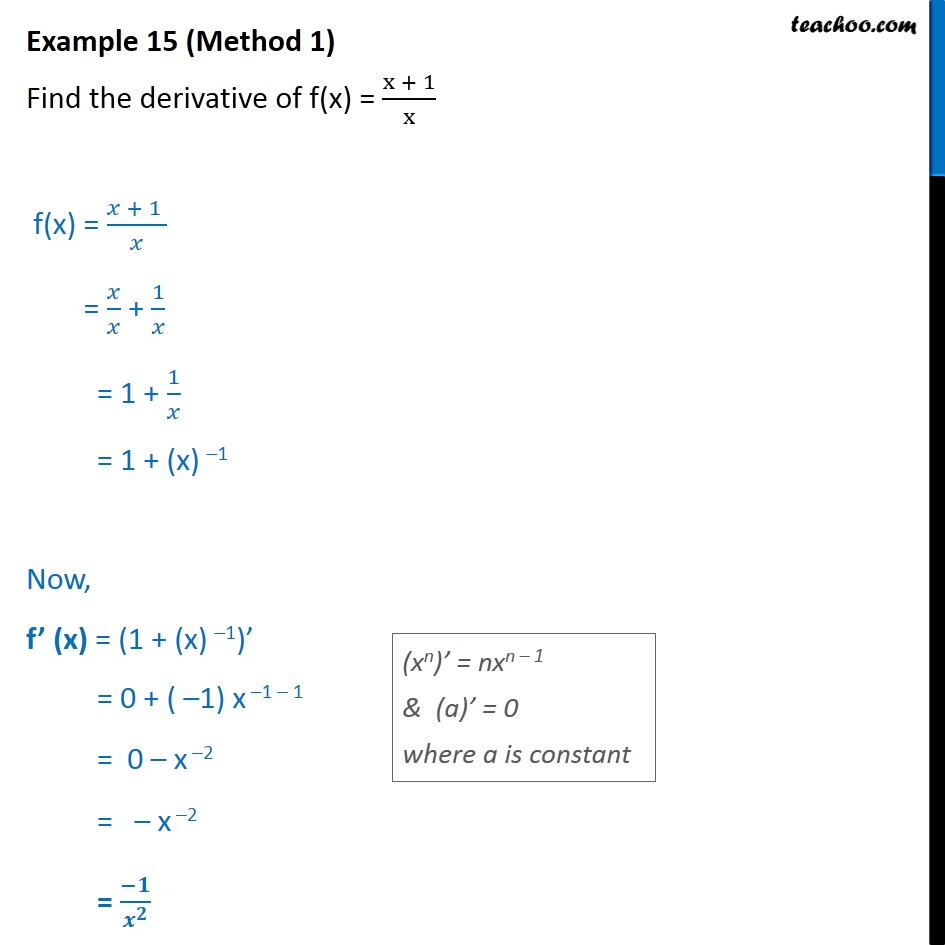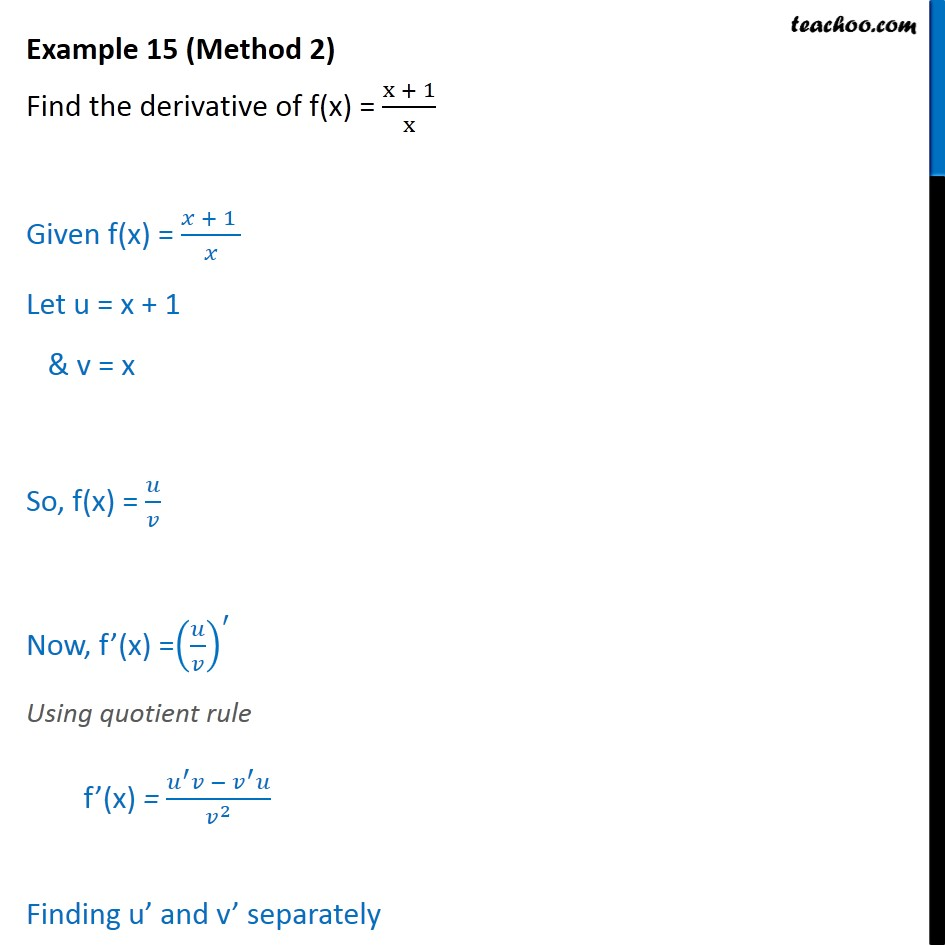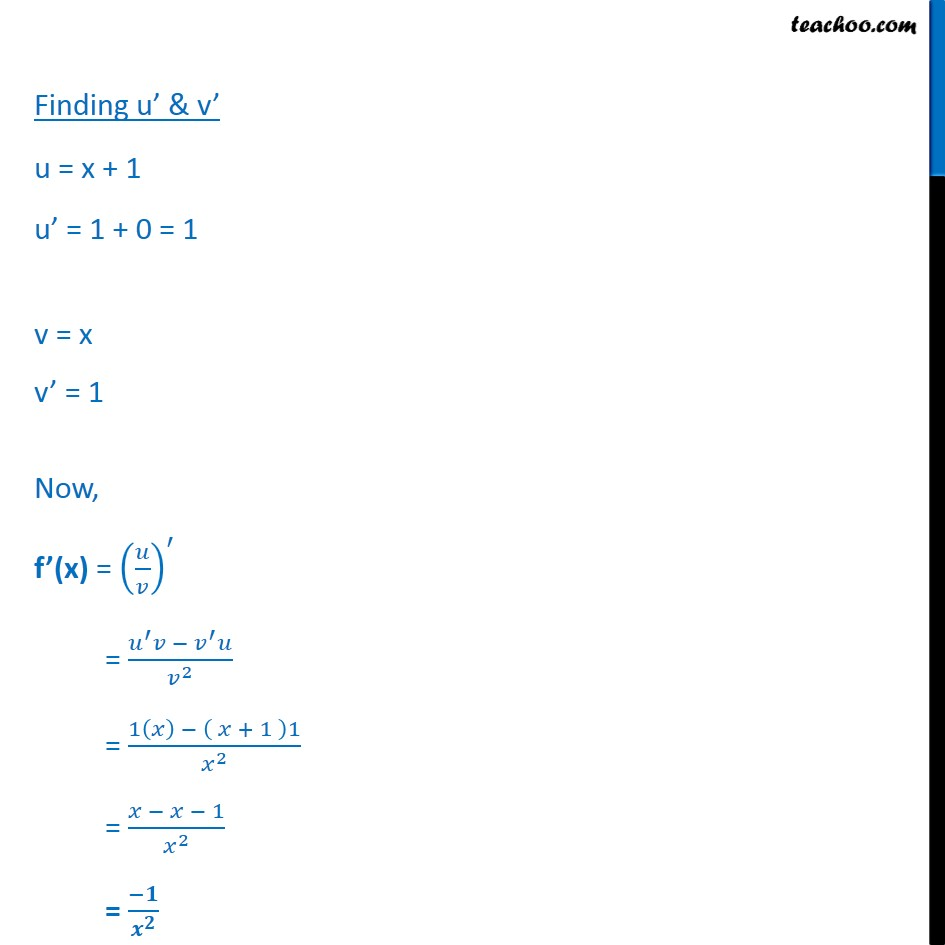1. Chapter 13 Class 11 Limits and Derivatives (Term 1 and Term 2)
2. Serial order wise
3. Examples (Term 1 and Term 2)

Transcript

Example 15 (Method 1) Find the derivative of f(x) = x + 1﷮x﷯ f(x) = 𝑥 + 1 ﷮𝑥﷯ = 𝑥﷮𝑥﷯ + 1﷮𝑥﷯ = 1 + 1﷮𝑥﷯ = 1 + (x) –1 Now, f’ (x) = (1 + (x) –1)’ = 0 + ( –1) x –1 – 1 = 0 – x –2 = – x –2 = −𝟏﷮ 𝒙﷮𝟐﷯﷯ Example 15 (Method 2) Find the derivative of f(x) = x + 1﷮x﷯ Given f(x) = 𝑥 + 1 ﷮𝑥﷯ Let u = x + 1 & v = x So, f(x) = 𝑢﷮𝑣﷯ Now, f’(x) = 𝑢﷮𝑣﷯﷯﷮′﷯ Using quotient rule f’(x) = 𝑢﷮′﷯𝑣 − 𝑣﷮′﷯𝑢﷮ 𝑣﷮2﷯﷯ Finding u’ and v’ separately Finding u’ & v’ u = x + 1 u’ = 1 + 0 = 1 v = x v’ = 1 Now, f’(x) = 𝑢﷮𝑣﷯﷯﷮′﷯ = 𝑢﷮′﷯𝑣 − 𝑣﷮′﷯𝑢﷮ 𝑣﷮2﷯﷯ = 1 𝑥﷯ − 𝑥 + 1 ﷯1﷮ 𝑥﷮2﷯﷯ = 𝑥 − 𝑥 + 1﷮ 𝑥﷮2﷯﷯ = 𝟏﷮ 𝒙﷮𝟐﷯﷯

Examples (Term 1 and Term 2)